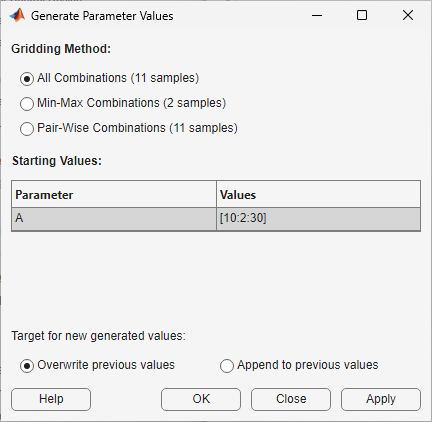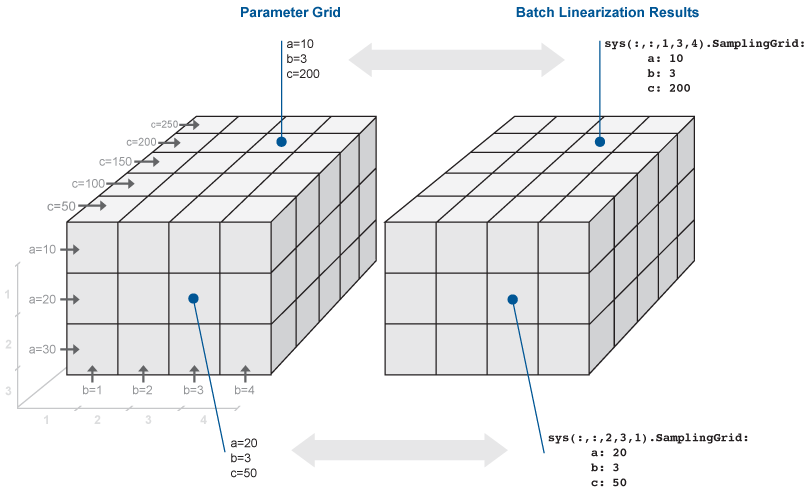## Specify Parameter Samples for Batch Linearization

Block parameters configure a Simulink® model in several ways. For example, you can use block parameters to specify various coefficients or controller sample times. You can also use a discrete parameter, like the control input to a Multiport Switch block, to control the data path within a model. Varying the value of a parameter helps you understand its impact on the model behavior.

When using any of the Simulink Control Design™ linearization tools (or tuning with `slTuner` or Control System Tuner) you can specify a set of block parameter values at which to linearize the model. The full set of values is called a parameter grid or parameter samples. The tools batch-linearize the model, computing a linearization for each value in the parameter grid. You can vary multiple parameters, thus extending the parameter grid dimension. When using the command-line linearization tools, the `linearize` command or the `slLinearizer` or `slTuner` interfaces, you specify the parameter samples using a structure with fields `Name` and `Value`. In the Model Linearizer or Control System Tuner, you use the graphical interface to specify parameter samples.

### Which Parameters Can Be Sampled?

You can vary any model parameter whose value is given by a variable in the model workspace, the MATLAB® workspace, or a data dictionary. In cases where the varying parameters are all tunable, the linearization tools require only one model compilation to compute transfer functions for varying parameter values. This efficiency is especially advantageous for models that are expensive to compile repeatedly.

For more information, see Batch Linearization Efficiency When You Vary Parameter Values.

### Vary Single Parameter at the Command Line

To vary the value of a single parameter for batch linearization with `linearize`, `slLinearizer`, or `slTuner`, specify the parameter grid as a structure having two fields. The `Name` field contains the name of the workspace variable that specifies the parameter. The `Value` field contains a vector of values for that parameter to take during linearization.

For example, the `Watertank` model has three parameters defined as MATLAB workspace variables, `a`, `b`, and `A`. The following commands specify a parameter grid for the single parameter for `A`.

```param.Name = 'A'; param.Value = Avals;```

Here, `Avals` is an array specifying the sample values for `A`.

The following table lists some common ways of specifying parameter samples.

Parameter Sample-Space TypeHow to Specify the Parameter Samples
Linearly varying`param.Value = linspace(A_min,A_max,num_samples)`
Logarithmically varying`param.Value = logspace(A_min,A_max,num_samples)`
Random`param.Value = rand(1,num_samples)`
Custom`param.Value = custom_vector`

If the variable used by the model is not a scalar variable, specify the parameter name as an expression that resolves to a numeric scalar value. For example, suppose that `Kpid` is a vector of PID gains. The first entry in that vector, `Kpid`, is used as a gain value in a block in your model. Use the following commands to vary that gain using the values given in a vector `Kpvals`:

```param.Name = 'Kpid(1)'; param.Value = Kpvals;```

After you create the structure `param`:

• Pass it to `linearize` as the `param` input argument.

• Pass it to `slLinearizer` as the `param` input argument, when creating an `slLinearizer` interface.

• Set the `Parameters` property of an existing `slLinearizer` interface to `param`.

### Vary Single Parameter in Graphical Tools

To specify variations of a single parameter for batch linearization in Model Linearizer, on the Linear Analysis tab, in the Parameter Variations drop-down list, click ```Select parameters to vary```. (In Control System Tuner, the Parameter Variations drop-down list is on the Control System tab.)ClickManage Parameters. In the Select model variables dialog box, check the parameter to vary. The table lists all variables in the MATLAB workspace and the model workspace that are used in the model, whether tunable or not.Note

If the parameter is not a scalar variable, click Specify expression indexing if necessary and enter an expression that resolves to a numeric scalar value. For example, if `A` is a vector, enter `A(3)` to specify the third entry in `A`. If `A` is a structure and the scalar parameter you want to vary is the `Value` field of that structure, enter `A.Value`. The indexed variable appears in the variable list.

ClickOK. The selected variable appears in the Parameter Variations table. Use the table to specify parameter values manually, or generate values automatically.

#### Manually Specify Parameter Values

To specify the values manually, add rows to the table by clickingInsert Row and selecting either ```Insert Row Above``` or `Insert Row Below`. Then, edit the values in the table as needed.When you return to the Linear Analysis tab and linearize the model, Model Linearizer linearizes at all parameter values listed in the Parameter Variations table.

Note

In Control System Tuner, when you are finished specifying your parameter variations, you must apply the changes before continuing with tuning. To do so, in the Parameter Variations tab, clickApply. Control System Tuner applies the specified parameter variations, relinearizes your model, and updates all existing plots.

#### Automatically Generate Parameter Values

To generate values automatically, clickGenerate Values. In the Generate Parameter Values dialog box, in the Values column, enter an expression for the parameter values you want for the variable. For example, enter an expression such as `linspace(A_min,A_max,num_samples)`, or `[10:2:30]`.ClickOverwrite to replace the values in the Parameter Variations table with the generated values.

When you return to the Linear Analysis tab and linearize the model, Model Linearizer computes a linearization for each of these parameter values.

Note

In Control System Tuner, when you are finished specifying your parameter variations, you must apply the changes before continuing with tuning. To do so, in the Parameter Variations tab, clickApply. Control System Tuner applies the specified parameter variations, relinearizes your model, and updates all existing plots.

### Multi-Dimension Parameter Grids

When you vary more than one parameter at a time, you generate parameter grids of higher dimension. For example, varying two parameters yields a parameter matrix, and varying three parameters yields a 3-D parameter grid. Consider the following parameter grid:Here, you vary the values of three parameters, a, b, and c. The samples form a 3-by-4-by-5 grid. When batch linearizing your model, the `ss` model array, `sys`, is the batch result. Similarly, when batch trimming your model, you get an array of operating point objects.

### Vary Multiple Parameters at the Command Line

To vary the value of multiple parameters for batch linearization with `linearize`, `slLinearizer`, or `slTuner`, specify parameter samples as a structure array. The structure has an entry for each parameter whose value you vary. The structure for each parameter is the same as described in Vary Single Parameter at the Command Line. You can specify the `Value` field for a parameter to be an array of any dimension. However, the size of the `Value` field must match for all parameters. Corresponding array entries for all the parameters, also referred to as a parameter grid point, must map to a desired parameter combination. When the software linearizes the model, it computes a linearization — an `ss` model — for each grid point. The software populates the `SamplingGrid` property of each linearized model with information about the parameter grid point that the model corresponds to.

#### Specify Full Grid

Suppose that your model has two parameters whose values you want to vary, a and b:

`$\begin{array}{l}a=\left\{a1,a2\right\}\\ b=\left\{b1,b2\right\}\end{array}$`

You want to linearize the model for every combination of a and b, also referred to as a full grid:

`$\left\{\begin{array}{cc}\left({a}_{1},{b}_{1}\right),& \left({a}_{1},{b}_{2}\right)\\ \left({a}_{2},{b}_{1}\right),& \left({a}_{2},{b}_{2}\right)\end{array}\right\}$`

Create a rectangular parameter grid using `ndgrid`.

```a1 = 1; a2 = 2; a = [a1 a2]; b1 = 3; b2 = 4; b = [b1 b2]; [A,B] = ndgrid(a,b) ```
```>> A A = 1 1 2 2 >> B B = 3 4 3 4```

Create the structure array, `params`, that specifies the parameter grid.

```params(1).Name = 'a'; params(1).Value = A; params(2).Name = 'b'; params(2).Value = B; ```

In general, to specify a full grid for N parameters, use `ndgrid` to obtain N grid arrays.

`[P1,...,PN] = ndgrid(p1,...,pN);`

Here, `p1`,...,`pN` are the parameter sample vectors.

Create a 1 x N structure array.

```params(1).Name = 'p1'; params(1).Value = P1; ... params(N).Name = 'pN'; params(N).Value = PN; ```

#### Specify Subset of Full Grid

If your model is complex or you vary the value of many parameters, linearizing the model for the full grid can become expensive. In this case, you can specify a subset of the full grid using a table-like approach. Using the example in Specify Full Grid, suppose you want to linearize the model for the following combinations of a and b:

`$\left\{\left({a}_{1},{b}_{1}\right),\left({a}_{1},{b}_{2}\right)\right\}$`

Create the structure array, `params`, that specifies this parameter grid.

```A = [a1 a1]; params(1).Name = 'a'; params(1).Value = A; B = [b1 b2]; params(2).Name = 'b'; params(2).Value = B; ```

### Vary Multiple Parameters in Graphical Tools

To vary the value of multiple parameters for batch linearization in Model Linearizer or Control System Tuner, open the Select model variables dialog box, as described in Vary Single Parameter in Graphical Tools. In the dialog box, check all variables you want to vary.Note

If a parameter you want to vary is not a scalar variable, click Specify expression indexing if necessary and enter an expression that resolves to a scalar value. For example, if `A` is a vector, enter `A(3)` to specify the third entry in `A`. If `A` is a structure and the scalar parameter you want to vary is the `Value` field of that structure, enter `A.Value`. The indexed variable appears in the variable list.

ClickOK. The selected variables appear in the Parameter Variations table. Each column in the table corresponds to one selected variable. Each row in the table represents one full set of parameter values at which to linearize the model. When you linearize, Model Linearizer computes as many linear models as there are rows in the table. Use the table to specify combinations of parameter values manually, or generate value combinations automatically.

#### Manually Specify Parameter Values

To specify the values manually, add rows to the table by clickingInsert Row and selecting either ```Insert Row Above``` or `Insert Row Below`. Then, edit the values in the table as needed. For example, the following table specifies linearization at four parameter-value pairs: `(Ki2,Kp2)` = (3.5,1), (3.5,2), (5,1), and (5,2).When you return to the Linear Analysis tab and linearize the model, Model Linearizer computes a linearization for each of these parameter-value pairs.

Note

In Control System Tuner, when you are finished specifying your parameter variations, you must apply the changes before continuing with tuning. To do so, in the Parameter Variations tab, clickApply. Control System Tuner applies the specified parameter variations, relinearizes your model, and updates all existing plots.

#### Automatically Generate Parameter Values

To generate values automatically, clickGenerate Values. In the Generate Parameter Values dialog box, in the Values column, enter an expression for the parameter values you want for each variable, such as `linspace(A_min,A_max,num_samples)`, or `[10:2:30]`. For example, the following entry generates parameter-value pairs for all possible combinations of ```Kp1 = [0.1,0.15,0.2,0.25,0.3]``` and ```Kp2 = [0.03,0.04,0.05]```.ClickOverwrite to replace the values in the Parameter Variations table with the generated values.

When you return to the Linear Analysis tab and linearize the model, Model Linearizer computes a linearization for each of these parameter-value pairs.

Note

In Control System Tuner, when you are finished specifying your parameter variations, you must apply the changes before continuing with tuning. To do so, in the Parameter Variations tab, clickApply. Control System Tuner applies the specified parameter variations, relinearizes your model, and updates all existing plots.